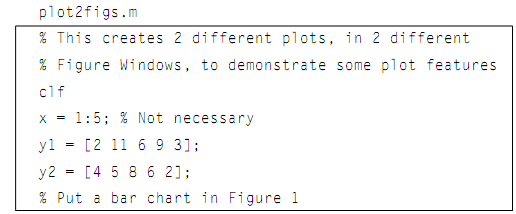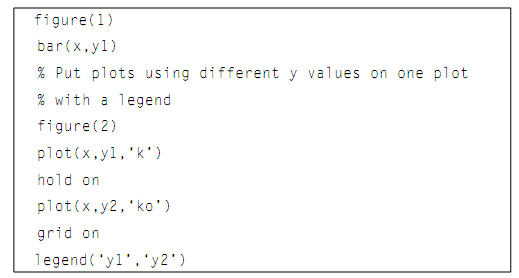## Illustration of grid function, C/C++ Programming

Assignment Help:

Illustration of Grid function:

For illustration, the script below creates two individual figure windows. At First, it clears the figure window. Then, it generates an x vector and two different y vectors (y1 & y2). In the first figure window, it plots the y1 values by using a bar chart. In the next figure window, it plots the y1 values as black lines, place hold on so that the next graph will be superimposed, and plots the y2 values as black o's. Also it puts a legend on this graph and uses a grid. The Labels and titles are absent in this case as it is generic data.#### Text Editor, Add a function for saving the text stored in an array to a fil...

Add a function for saving the text stored in an array to a file. Your program must check whether or not the output file already exists, and if it does, your program must ask the us

#### # Bank Account class, Write a C++ program to test the Bank Account class fo...

Write a C++ program to test the Bank Account class for 10 customers.

#### Area under curve, Write a program to find the area under the curve y = f(x)...

Write a program to find the area under the curve y = f(x) between x = a and x = b, integrate y = f(x) between the limits of a and b. The area under a curve between two points can b

#### The Shell or Command Line Interpreter is the fundamental Use, 1. The shell ...

1. The shell must support the following internal commands: i. cd - Change the current default directory to . If the argument is not present, report the current directory. If t

#### Last fibonnaci standing, i need a program for finding the last fibonacci nu...

i need a program for finding the last fibonacci number

#### Stand-alone dsp application, You are required to write a stand-alone DSP ap...

You are required to write a stand-alone DSP application in the C programming language. The overall objective of this application is to convert a signal from the time domain to t

#### Define bitwise-shift operators, Define Bitwise-Shift Operators? The shi...

Define Bitwise-Shift Operators? The shift operators perform suitable shift by operator on the right to the operator on the left. The right operator should be positive. The va

#### program to conduct timber structural component design, The question descri...

The question description: You are required to make a C++ program to conduct timber component (beam and column) structural design according to EC5 (all the relevant design codes

#### Area under curve, Write a program to find the area under the curve y = f(x)...

Write a program to find the area under the curve y = f(x) between x = a and x = b, integrate y = f(x) between the limits of a and b.

#### Pointers, Pointers are just numbers, representing addresses in memory. You ...

Pointers are just numbers, representing addresses in memory. You can add to and subtract from pointers, for instance. It is pretty easy to make mistakes with pointer math, however,